# Array helpers for Javascript

I would like to represent you some useful Javascript helper functions for manipulating data.

## .forEach()

forEach() calls a provided callback function once for each element in an array in ascending order.

Syntax:

`arr.forEach(callback(currentValue[, index[, array]]) {  // execute something}[, thisArg]);`

Example: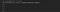forEach() function

## .filter()

flter() creates a new array with all elements that pass the test implemented by the provided function.

Syntax:

`let newArray = arr.filter(callback(currentValue[, index[, array]]) {  // return element for newArray, if true}[, thisArg]);`

Example: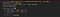filter() function

## .find()

find() returns the value of the first element in the provided array that satisfies the provided condition.

Syntax:

`arr.find(callback(element[, index[, array]])[, thisArg])`

Example: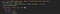find() function

## .map()

map() creates a new array with all elements that pass the test implemented by the provided function.

Syntax:

`let newArray = arr.map(callback(currentValue[, index[, array]]) {  // return element for newArray, after executing something}[, thisArg]);`

Example: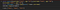map() function

## .reduce()

reduce() executes a reducer dunction (that you provide) on each element of the array, resulting in single output value.

Syntax:

`arr.reduce(callback( accumulator, currentValue, [, index[, array]] )[, initialValue])`

Example: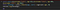reduce() function